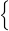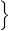Sa T.

# Let S = {d, g, k, r, u, x} be a sample space of an experiment

Let S = {dgkrux} be a sample space of an experiment and let E = {dg} and F = {drx} be events of this experiment. (Enter ∅ for the empty set.)

Find the events E ∪ F and E ∩ F.

 E ∪ F =E ∩ F =